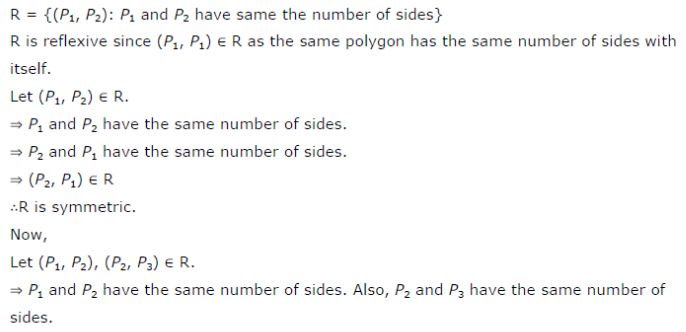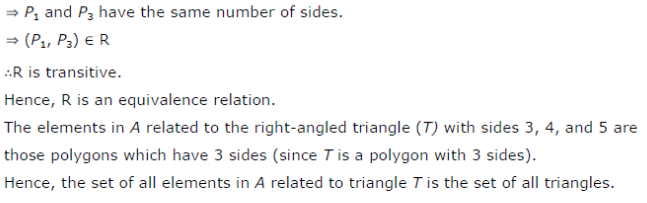# Show that the relation R defined in the set A of all polygons as R = {(P1, P2) : P1 and P2

Show that the relation R defined in the set A of all polygons as R = {(P1, P2) : P1 and P2 have same number of sides}, is an equivalence relation. What is the set of all elements in A related to the right angle triangle T with sides 3, 4, and 5?